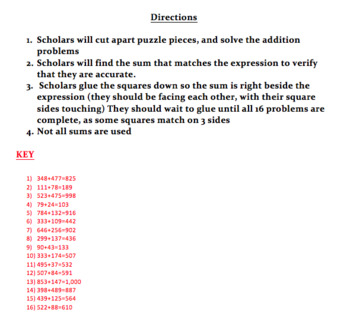Subject
Resource Type
File Type
Word Document File (1 MB|3 pages)
Standards
\$1.50
• Product Description
• Standards

This is a puzzle for scholars to practice addition within 1,000. Your purchase comes with the puzzle pieces, a key for the problems, directions, and a picture for an example.

Directions:

1. Scholars cut out the 16 puzzle pieces, which include 16 addition problems

2. Scholars match the expressions up to the proper sum, if they cannot find the sum they have made an error.

3. Scholars should glue the pieces down at the end, I would not let them do it as they work as some problems match on 3 sides (not all sums will be used).

There is a version of this puzzle that includes only subtraction here: https://www.teacherspayteachers.com/Product/Subtraction-Within-1000-Puzzle-3835031

Fluently add and subtract multi-digit whole numbers using the standard algorithm.
Fluently add and subtract within 1000 using strategies and algorithms based on place value, properties of operations, and/or the relationship between addition and subtraction.
Add and subtract within 1000, using concrete models or drawings and strategies based on place value, properties of operations, and/or the relationship between addition and subtraction; relate the strategy to a written method. Understand that in adding or subtracting three-digit numbers, one adds or subtracts hundreds and hundreds, tens and tens, ones and ones; and sometimes it is necessary to compose or decompose tens or hundreds.
Total Pages
3 pages